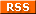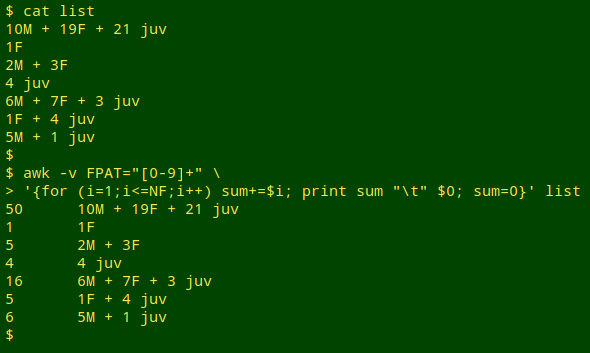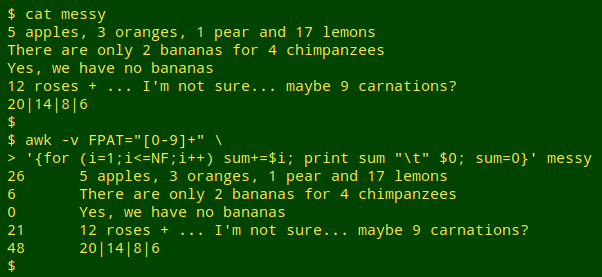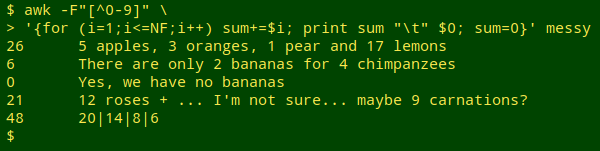For a full list of BASHing data blog posts, see the index page.# How many fruits in 5 apples, 3 oranges, 1 pear and 17 lemons?

I have a list of tallies of millipede specimens, like this but much longer:

10M + 19F + 21 juv
1F
2M + 3F
4 juv
6M + 7F + 3 juv
1F + 4 juv
5M + 1 juv

and I want the total number of individuals in each tally.

One solution is to use the AWK option "FPAT" (GNU AWK 4). Most of the time I write code that first tells AWK what the field separators are in a record, for example a comma (-F",") or a tab (-F"\t"). However, I can also tell AWK what the fields themselves are, if I specify their nature with a regular expression.

In the code below, I describe each field with FPAT as 1 or more digits ([0-9]+). I then tell AWK to go through each line field by field, adding the number in each field to the variable "sum", then printing "sum", a tab and the whole line, then resetting "sum" to zero before going on to the next line.

awk -v FPAT="[0-9]+" '{for (i=1;i<=NF;i++) sum+=\$i; print sum "\t" \$0; sum=0}' list"sum" could also be reset to an empty string (sum="").

In my millipede tallies, "M" means male, "F" means female and "juv" means "juvenile". With this FPAT setting, AWK doesn't care — it only looks at the numbers:Turning the first solution on its head, I can also do the sum if I tell AWK that the field separators can be anything that is not a number (-F"[^0-9]"):but I prefer "straight logic" to "inverse logic".

Last update: 2018-12-13
The blog posts on this website are licensed under a